## Selina Concise Mathematics Class 10 ICSE Solutions Chapter 10 Arithmetic Progression Ex 10E

These Solutions are part of Selina Concise Mathematics Class 10 ICSE Solutions. Here we have given Selina Concise Mathematics Class 10 ICSE Solutions Chapter 10 Arithmetic Progression Ex 10E

Other Exercises

Question 1.
Two cars start together in the same direction from the same place. The first car goes at uniform speed of 10 km h-1. The second car goes at a speed of 8 km h-1 in the first hour and thereafter increasing the speed by 0.5 km h-1 each succeeding hour. After how many hours will the two cars meet ?
Solution:
Speed of first car = 10 km/hr
Speed of second car = 8 km/hr in first hour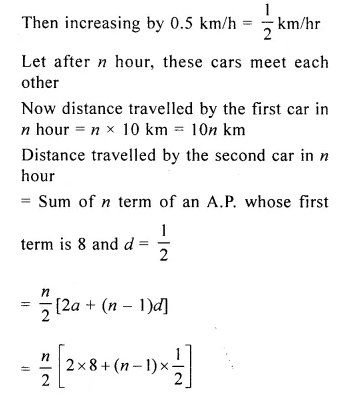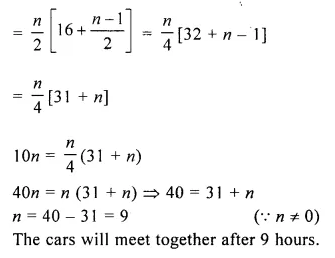Question 2.
A sum of Rs 700 is to be paid to give seven cash prizes to the students of a school for their overall academic performance. If the cost of each prize is Rs 20 less than its preceding prize; find the value of each of the prizes.
Solution:
Total amount (Sn) = Rs 700
Cost of each prize is Rs 20 less than its preceding price or d = – 20
d = – 20 and n = 7
$${ S }_{ n }=\frac { n }{ 2 } \left[ 2a+(n-1)d \right]$$Question 3.
An article can be bought by paying Rs 28,000 at once or by making 12 monthly installments. If the first installment paid is Rs 3,000 and every other installment is Rs 100 less than the previous one, find :
(i) amount of installment paid in the 9th month
(ii) total amount paid in the installment scheme.
Solution:
Total price of an article = Rs 28000
No. of installments (n) = 12
First installment (a) = RS 3000
d = Rs 100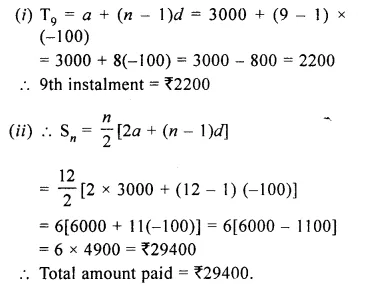Question 4.
A manufacturer of TV sets produces 600 units in the third year and 700 units in the 7th year. Assuming that the production increases uniformly by a fixed number every year, find :
(i) the production in the first year.
(ii) the production in the 10th year.
(iii) the total production in 7 years.
Solution:
A manufacture of TV sets, he produces
No. of units in 3rd year = 600
No. of units in 7th year = 700
Let a be the first term and d be the common difference, then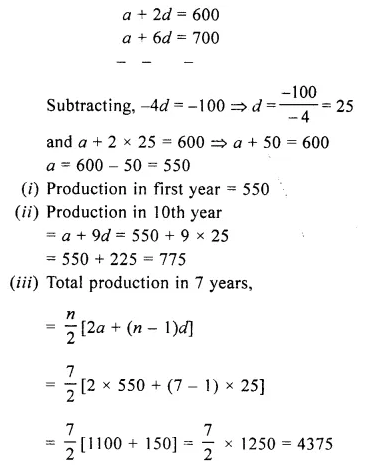Question 5.
Mrs. Gupta repays her total loan of Rs 1.18,000 by paying installments every month. If the installment for the first month is Rs 1,000 and it increases by RS 100 every month, what amount will she pay as the 30th installment of loan? What amount of loan she still has to pay after the 30th installment?
Solution:
Total loan to be paid by Mrs. Gupta = Rs 118000
Installment for the first month (a) = Rs 1000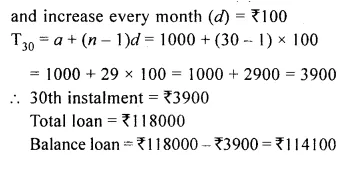Question 6.
In a school, students decided to plant trees in and around the school to reduce air pollution. It was decided that the number of trees, that each section of each class will plant, will be five times of the class to which the respective section belongs. If there are 1 to 10 classes in the school and each class has three sections, find how many trees were planted by the students?
Solution:
Number of classes = 10
Number of sections of each class = 3
Total number of sections = 10 x 3 = 30
Each section plant tree = 5 times of the class
Each section of 1st class will plant = 1 x 15 = 15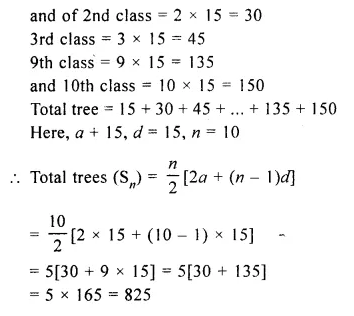Hope given Selina Concise Mathematics Class 10 ICSE Solutions Chapter 10 Arithmetic Progression Ex 10E are helpful to complete your math homework.

If you have any doubts, please comment below. Learn Insta try to provide online math tutoring for you.